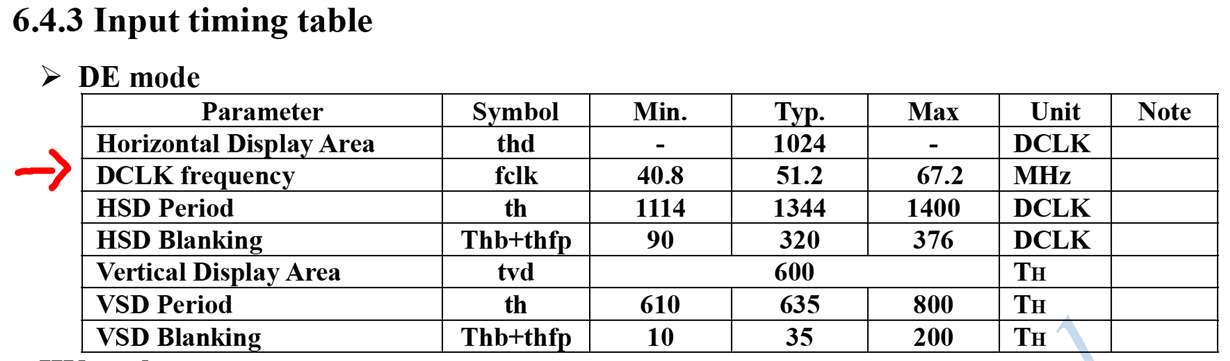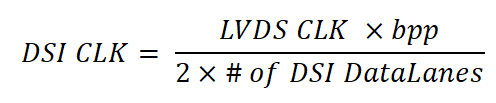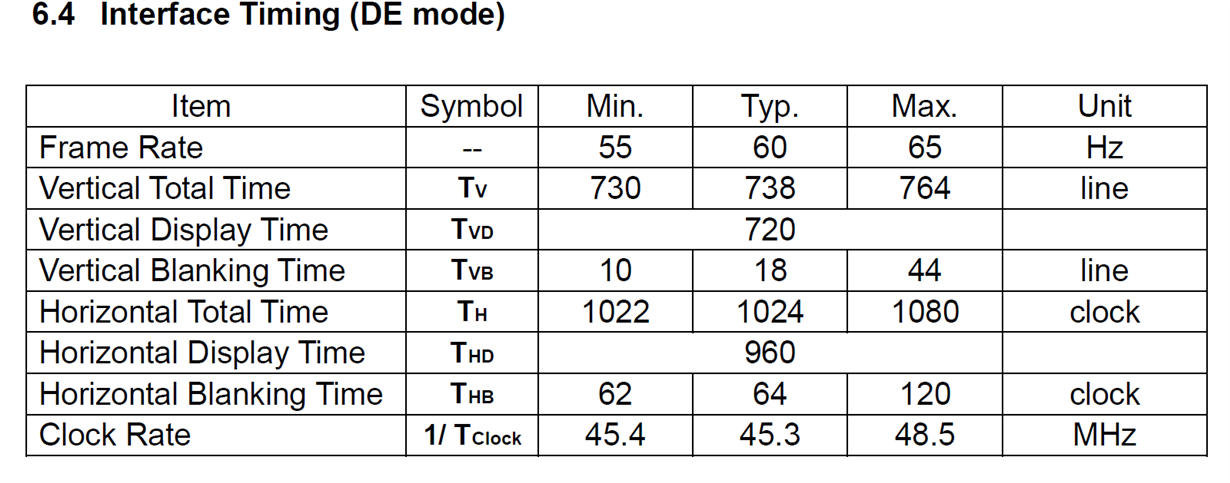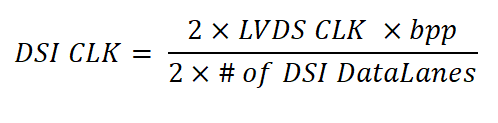If you have a related question, please click the "Ask a related question" button in the top right corner. The newly created question will be automatically linked to this question.

• Resolved

# [FAQ] SN65DSI84: SN65DSI83, SN65DSI84, and SN65DSI85 resolution guide

Part Number: SN65DSI84

Will the SN65DSI83, SN65DSI84, or SN65DSI85 support my display panel resolution?

• The way to know whether or not the SN65DSI83, SN65DSI84, or SN65DSI85 will support your display panel resolution is by determining what LVDS clock frequency your display panel needs. If you have the datasheet for the display panel then this is very easy to find, as there should be a table that shows all the timing specifications.

Single-channel DSI to single-channel LVDS (SN65DSI83)

For example, for a single-channel LVDS display, the table will look something like the below:From the above you can see that the typical clock frequency the display needs is 51.2 MHz.

The SN65DSI83’s output clock frequency range is 25 – 154 MHz. Since the display panel’s frequency requirement is within range of the SN65DSI83’s supported clock frequencies, this display panel can be supported.

Now, what about the DSI CLK? To determine what DSI CLK is needed for a single-channel DSI to single-channel LVDS application, you will use this equation:Using the previously provided 51.2 MHz as the LVDS CLK frequency, let’s also assume that the panel is 24-bpp (4 LVDS data lanes being used) and that all 4 DSI data lanes on the SN65DSI83 will be used. Plugging all of these into the equation will give a DSI CLK frequency of 153.6 MHz. The DSI CLK range supported by the SN65DSI83 is 40 – 500 MHz, so this is fine.

Now, what do you do if you don’t have your display panel datasheet yet? In this case, if you at least know what resolution you want to support then you can estimate what LVDS CLK frequency will be required using the equation below:As an example, let’s say that the resolution you want to support is 1920x1080@60Hz

• HActive = 1920
• VActive = 1080
• Frame rate = 60
• As a rule of thumb we can estimate the %blanking to be 20% (0.2)

Plugging these numbers into the equation, you can estimate the LVDS CLK frequency to be 149.3 MHz, which is within range of the SN65DSI83’s supported frequencies. So we can assume that the SN65DSI83 will be able to support a 1920x1080@60Hz display panel, but you will still want to verify this once you are able to get the datasheet for the display panel.

• In reply to I.K. Anyiam:

Single-channel DSI to dual-channel LVDS (SN65DSI84)

For single-channel DSI to dual-channel LVDS with the SN65DSI84, the idea is very similar.

If you have the datasheet for your dual LVDS display you can find what clock frequency it requires in the timing section like below:However, note that with dual LVDS displays there are 2 LVDS clocks. Typically the datasheet will only give the frequency of 1 of the clocks like the above. The other clock will have the same frequency though, so for this example both LVDS clocks require 45.3 MHz. Both LVDS clock outputs on the SN65DSI84 support frequencies in the range of 25 MHz to 154 MHz so this display panel will be supported.

The DSI CLK frequency requirement for a single-channel DSI to dual-channel LVDS application is similar to that of a single-channel DSI to single-channel LVDS application with one difference. Remember that there are 2 output clocks now instead of 1, and both clocks need to be taken into consideration for the DSI CLK since the DSI CLK will need to support both. So the equation is now:Using the previously provided 45.3 MHz as the LVDS CLK frequency, let’s also assume that the panel is 24-bpp (8 LVDS data lanes being used – 4 data lanes per LVDS clock) and that all 4 DSI data lanes on the SN65DSI84 will be used. Plugging all of these into the equation will give a DSI CLK frequency of 271.8 MHz. The DSI CLK range supported by the SN65DSI84 is 40 – 500 MHz, so this is fine.

Now, what do you do if you don’t have your display panel datasheet yet? You can still estimate what frequency you will need if you know what resolution you want to support. Let’s take 1920x1080@60Hz as an example resolution again. We can use the same equation from the single-channel DSI to single-channel LVDS section, except we can divide it by 2 since there are 2 LVDS clocks. This equation will give the estimated frequency of each LVDS clock for a dual channel LVDS display:So assuming we have 20% blanking again, plugging our numbers in we get an estimated frequency of 74.65 MHz for each LVDS CLK. This is within range of the LVDS CLK supported frequencies. If we plug this into the DSI CLK equation (assuming 24bpp and 4 DSI data lanes being used) the required DSI CLK frequency is 447.9 MHz, which is within range of the SN65DSI84’s supported DSI CLK frequency range.

• In reply to I.K. Anyiam:

Dual-channel DSI to dual-channel LVDS (SN65DSI85)

For this we can use the same examples as the single-channel DSI to dual-channel LVDS section since they both use dual LVDS displays:As before, this will be supported by the SN65DSI85 since both clocks can support frequencies within 25 – 154 MHz. The DSI CLK frequency equation will also be the same as in the single-channel DSI to dual-channel LVDS section since there are 2 LVDS clocks:However, there are now 2 DSI CLKs and more DSI data lanes. For a dual-channel DSI to dual-channel LVDS application it’s safe to assume that you’ll be using all 8 DSI data lanes on the SN65DSI85. In this case, for a 24bpp application, plugging our numbers into the above equation, each DSI CLK will need to be 135.9 MHz, which is within range of the SN65DSI85’s DSI CLK supported frequencies.

For estimating the frequency without having the display panel datasheet, the process is similar to the previous sections. Using 1920*1080@60Hz, we can use the same equation as in the single-channel DSI to dual-channel LVDS section:So assuming we have 20% blanking again, plugging our numbers in we get an estimated frequency of 74.65 MHz for each LVDS CLK. This is within range of the LVDS CLK supported frequencies. If we plug this into the DSI CLK equation (assuming 24bpp and 8 DSI data lanes being used) the required DSI CLK frequency is 223.95 MHz for each clock, which is within range of the SN65DSI85’s supported DSI CLK frequency range.